music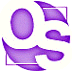# floating point numbers

## summary

Floating point numbers are used to roughly approximate real numbers.

It is important to remember that computer floating point numbers are usually a rough approximation of actual real numbers and are therefore subject to a wide variety of errors.

## free computer programming text book project

If you like the idea of this project,### stub section

This subchapter is a stub section. It will be filled in with instructional material later. For now it serves the purpose of a place holder for the order of instruction.

Professors are invited to give feedback on both the proposed contents and the propsed order of this text book. Send commentary to Milo, PO Box 1361, Tustin, California, 92781, USA.

# floating point numbers

Floating point numbers are used to roughly approximate real numbers.

It is important to remember that computer floating point numbers are usually a rough approximation of actual real numbers and are therefore subject to a wide variety of errors.

Alan Turing’s famous 1936 paper “On Computable Numbers, with an Application to the Entscheidungsproblem” imagined Turing Machines capable of creating infinite strings of binary digits, thereby representing real numbers. He famously proved that only a subset of all real numbers could be computed by a machine. These are the computable numbers, which include the integers, the rational numbers (which include integers), the algebriac numbers (which include integers, rational numbers, and irrational numbers that are roots of rational numbers), and some transcendental numbers, such as π, e, trigonometric functions, logarithmic functions, and the real parts of the zeros of the Bessel functions.

Turing famously proved that the vast majority of all possible real numbers can not be computed.

Because physical computers are limited to finite time and memory, they can produce an even smaller fraction of possible computable numbers.

The important point here is that floating point numbers are approximations, not the actual numbers they supposedly represent, so they almost always start with some small error. As you manipulate these numbers (such as add, subtract, multiply, and divide), you increase the amount of error in your computations.

Usually the errors are small and can be safely ignored, but under the wrong conditions the errors can become huge in a hurry, producing garbage results. A surprising number of professional programmers get caught by this “gothca” every year.

### floating point type

Most programming languages have a floating point type. This is a computer representation of the mathematical real numbers.

Unlike mathematical real numbers, computer floating point numbers have a range, a maximum (largest) and minimum (smallest negative) number.

## JOVIAL

The following material is from the unclassified Computer Programming Manual for the JOVIAL (J73) Language, RADC-TR-81-143, Final Technical Report of June 1981.

The kinds of values provided by JOVIAL reflect the applications
of the language; they are oriented toward engineering and contrl
programming rather than, for example, commercial and business
programming.  The JOVIAL values are:
2.  Floating values, which are numbers with "floating" scale
factors.  They are used for physical quantities,
especially when the range of measurement cannot be
accurately predicted.  For example, floating values are
frequently used to represent distance, speed,
temperature, time, and so on.

Chapter 1 INTRODUCTION, page 2

ITEM SPEED F 30;      A floating item, whose value is stored
as a variable coefficient (mantissa)
and variable scale factor (exponent).
The "30" specifies thirty bits for the
mantissa and thus determines the
accuacy of the value.  The number of
bits in the exponent is specified by
the implementation, not the program.
It is always sufficient to accommodate
a wide range of numbers.

Chapter 1 INTRODUCTION, page 4

## ALGOL 68

In ALGOL 68 the floating point mode is declared with the reserved word real.

real    FinalAverage;

## Pascal

In Pascal the floating point type is declared with the reserved word real.

var    FinalAverage: real;

## C

In C the floating point type is declared with the key word float.

float FinalAverage;

## Stanford essentials

Stanford CS Education Library This [the following section until marked as end of Stanford University items] is document #101, Essential C, in the Stanford CS Education Library. This and other educational materials are available for free at http://cslibrary.stanford.edu/. This article is free to be used, reproduced, excerpted, retransmitted, or sold so long as this notice is clearly reproduced at its beginning. Copyright 1996-2003, Nick Parlante, nick.parlante@cs.stanford.edu.

### Floating point Types

 float Single precision floating point number typical size: 32 bits double Double precision floating point number typical size: 64 bits long double Possibly even bigger floating point number (somewhat obscure)

Constants in the source code such as 3.14 default to type double unless the are suffixed with an ‘f’ (float) or ‘l’ (long double). Single precision equates to about 6 digits of precision and double is about 15 digits of precision. Most C programs use double for their computations. The main reason to use float is to save memory if many numbers need to be stored. The main thing to remember about floating point numbers is that they are inexact. For example, what is the value of the following double expression?

(1.0/3.0 + 1.0/3.0 + 1.0/3.0)    // is this equal to 1.0 exactly?

The sum may or may not be 1.0 exactly, and it may vary from one type of machine to another. For this reason, you should never compare floating numbers to each other for equality (==) -- use inequality (<) comparisons instead. Realize that a correct C program run on different computers may produce slightly different outputs in the rightmost digits of its floating point computations.

Stanford CS Education Library This [the above section] is document #101, Essential C, in the Stanford CS Education Library. This and other educational materials are available for free at http://cslibrary.stanford.edu/. This article is free to be used, reproduced, excerpted, retransmitted, or sold so long as this notice is clearly reproduced at its beginning. Copyright 1996-2003, Nick Parlante, nick.parlante@cs.stanford.edu.

## PL/I

Float Decimal declarations:

type of data: coded arithmetic

S/360, S/370 data format: floating point

default precision: six (6) decimal digits

maximum precision: 16 decimal digits
33 decimal digits for OS PL/I Optimizing Compiler

range of exponent: 10-78 to 10+75

example:

DECLARE LIGHT_YEARS FLOAT DECIMAL (16) INIT (3.1415E+20);

May be initialized with either fixed point decimals or floating point decimals.

Most useful for scientific processing requiring very large or very small numbers. The fractional part of floating point numbers are not exact.

Float Binary declarations:

type of data: coded arithmetic

S/360, S/370 data format: floating point

default precision: 21 binary bits

maximum precision: 53 binary bitsdigits
109 binary bits for OS PL/I Optimizing and Checkout Compilers

range of exponent: 2-260 to 2+252

example:

DECLARE LIGHT_YEARS FLOAT BINARY (53) INIT (1911E+54B);

The FLOAT DECIMAL and FLOAT BINARY are stored in memry in the exact same format. The FLOAT BINARY declaration is provided for programmers who want to control the exact number of binary bits used.

## Ruby

There are no data types in Ruby. Instead there are objects, as Ruby is exclusively an Object Oriented Programming language.

Ruby’s base class for numbers is Numeric.

Ruby’s numeric class Float holds floating-point numbers, using the underlying native machine double-precsision floating-point representation.

### floating point notation

The floating point number is often input and output in a floating point notation, a variation of scientific notation.

The floating point number is often input and output in a floating point notation, a variation of scientific notation.

The format (from left to right) is a sign for the mantissa, the mantiassa (which may have a decimal point and both an integer and fractional part), the letter E, a positive or negative sign for the exponent, and the exponent (which may have a decimal point and both an integer and fractional part).

In the vast majority of languages the fractional part is optional, but if there is a fractional part then there must be at least one digit to the left of the decimal point (although it can be a zero).

In many languages it is possible to leave off the exponent part.

Examples:

notation number
0.0 0
0.5 0.5
(half)
-1.23 -1.23
negative
5E+7 50000000
50,000,000
5.5E+7 55000000
55,000,000
5.5E-04 0.00055
-0.000255E+05 25.5

“31 Every object in the language has a type, which characterizes a set of values and a set of applicable operations. The main classes of types are elementary types (comprising enumeration, numeric, and access types) and composite types (including array and record types).” —:Ada-Europe’s Ada Reference Manual: Introduction: Language Summary See legal information

“33 Numeric types provide a means of performing exact or approximate numerical computations. Exact computations use integer types, which denote sets of consecutive integers. Approximate computations use either fixed point types, with absolute bounds on the error, or floating point types, with relative bounds on the error. The numeric types Integer, Float, and Duration are predefined.” —:Ada-Europe’s Ada Reference Manual: Introduction: Language Summary See legal information

### floating point representations

Floating point numbers are the computer equivalent of “scientific notation” or “engineering notation”. A floating point number consists of a fraction (binary or decimal) and an exponent (bianry or decimal). Both the fraction and the exponent each have a sign (positive or negative).

In the past, processors tended to have proprietary floating point formats, although with the development of an IEEE standard, most modern processors use the same format. Floating point numbers are almost always binary representations, although a few early processors had (binary coded) decimal representations. Many processors (especially early mainframes and early microprocessors) did not have any hardware support for floating point numbers. Even when commonly available, it was often in an optional processing unit (such as in the IBM 360/370 series) or coprocessor (such as in the Motorola 680x0 and pre-Pentium Intel 80x86 series).

Hardware floating point support usually consists of two sizes, called single precision (for the smaller) and double precision (for the larger). Usually the double precision format had twice as many bits as the single precision format (hence, the names single and double). Double precision floating point format offers greater range and precision, while single precision floating point format offers better space compaction and faster processing.

F_floating format (single precision floating), DEC VAX, 32 bits, the first bit (high order bit in a register, first bit in memory) is the sign magnitude bit (one=negative, zero=positive or zero), followed by 15 bits of an excess 128 binary exponent, followed by a normalized 24-bit fraction with the redundant most significant fraction bit not represented. Zero is represented by all bits being zero (allowing the use of a longword CLR to set a F_floating number to zero). Exponent values of 1 through 255 indicate true binary exponents of -127 through 127. An exponent value of zero together with a sign of zero indicate a zero value. An exponent value of zero together with a sign bit of one is taken as reserved (which produces a reserved operand fault if used as an operand for a floating point instruction). The magnitude is an approximate range of .29*10-38 through 1.7*1038. The precision of an F_floating datum is approximately one part in 223, or approximately seven (7) decimal digits).

32 bit floating format (single precision floating), AT&T DSP32C, 32 bits, the first bit (high order bit in a register, first bit in memory) is the sign magnitude bit (one=negative, zero=positive or zero), followed by 23 bits of a normalized two’s complement fractional part of the mantissa, followed by an eight bit exponent. The magnitude of the mantissa is always normalized to lie between 1 and 2. The floating point value with exponent equal to zero is reserved to represent the number zero (the sign and mantissa bits must also be zero; a zero exponent with a nonzero sign and/or mantissa is called a “dirty zero” and is never generated by hardware; if a dirty zero is an operand, it is treated as a zero). The range of nonzero positive floating point numbers is N = [1 * 2-127, [2-2-23] * 2127] inclusive. The range of nonzero negative floating point numbers is N = [-[1 + 2-23] * 2-127, -2 * 2127] inclusive.

40 bit floating format (extended single precision floating), AT&T DSP32C, 40 bits, the first bit (high order bit in a register, first bit in memory) is the sign magnitude bit (one=negative, zero=positive or zero), followed by 31 bits of a normalized two’s complement fractional part of the mantissa, followed by an eight bit exponent. This is an internal format used by the floating point adder, accumulators, and certain DAU units. This format includes an additional eight guard bits to increase accuracy of intermediate results.

D_floating format (double precision floating), DEC VAX, 64 bits, the first bit (high order bit in a register, first bit in memory) is the sign magnitude bit (one=negative, zero=positive or zero), followed by 15 bits of an excess 128 binary exponent, followed by a normalized 48-bit fraction with the redundant most significant fraction bit not represented. Zero is represented by all bits being zero (allowing the use of a quadword CLR to set a D_floating number to zero). Exponent values of 1 through 255 indicate true binary exponents of -127 through 127. An exponent value of zero together with a sign of zero indicate a zero value. An exponent value of zero together with a sign bit of one is taken as reserved (which produces a reserved operand fault if used as an operand for a floating point instruction). The magnitude is an approximate range of .29*10-38 through 1.7*1038. The precision of an D_floating datum is approximately one part in 255, or approximately 16 decimal digits).

### floating point registers

Floating point registers are special registers set aside for floating point math.

### history

Floating point arithmetic was first proposed independently by Leonardo Torres y Quevedo in Madrid in 1914, by Konrad Zuse in Berlin in 1936, and by George Stibitz in New Jersey in 1939. Zuse built floating point hardware that he called “semi-logarithmic notation” and included the ability to handle infinity and undefined. The first American computers with floating point hardware were the Bell Laboratories’ Model V and the Harvard Mark II in 1944 (relay computers).

# free music player coding example

Coding example: I am making heavily documented and explained open source code for a method to play music for free — almost any song, no subscription fees, no download costs, no advertisements, all completely legal. This is done by building a front-end to YouTube (which checks the copyright permissions for you).

View music player in action: www.musicinpublic.com/.

Create your own copy from the original source code/ (presented for learning programming).

## view text bookHTML file

Because I no longer have the computer and software to make PDFs, the book is available as an HTML file, which you can convert into a PDF.

 Tweets by @osdata

## free computer programming text book project

Building a free downloadable text book on computer programming for university, college, community college, and high school classes in computer programming.

If you like the idea of this project,

send donations to:
Milo
PO Box 1361
Tustin, California 92781

Supporting the entire project:

If you have a business or organization that can support the entire cost of this project, please contact Pr Ntr Kmt (my church)

Some or all of the material on this web page appears in the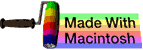This web site handcrafted on Macintosh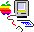computers using Tom Bender’s Tex-Edit Plus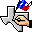and served using FreeBSD.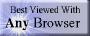†UNIX used as a generic term unless specifically used as a trademark (such as in the phrase “UNIX certified”). UNIX is a registered trademark in the United States and other countries, licensed exclusively through X/Open Company Ltd.

Names and logos of various OSs are trademarks of their respective owners.